# Geometric Integration

## Who am I?

• Olivier (Olle) Verdier
• French, Swedish
• PhD in Lund (2009)
• Postdocs in Cologne, Trondheim, Bergen, Umeå
• Future associate professor at HiB

## Book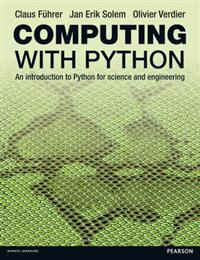## This talk

Collaborators

McLachlan        Munthe-Kaas        Modin

Talk available at olivierverdier.com/s/hib-2014-10

# Part 1: Polymorphism

## Polymorphism

Length of a list (of String)

length :: [String] -> Int

What about length of list of Double?:

length :: [Double] -> Int

Polymorphism:

length :: forall a. [a] -> Int

Important: No type introspection

## Type specification

Find a function with the type specification

f :: forall a. a -> a

One possibility

f x = x

It is the only possibility

...but how to prove this?

### “No type introspection”?

[Reynolds '83]Choose arbitrary relation $$\simeq \subset A_1\times A_2$$

$$x_1 \colon A_1$$, $$x_2\colon A_2$$, $$x_1 \simeq x_2\implies f(x_1) \simeq f(x_2)$$

So $$f \colon \forall A. A \to A$$ preserves all relations!

### Differential Equation

[Newton 1671]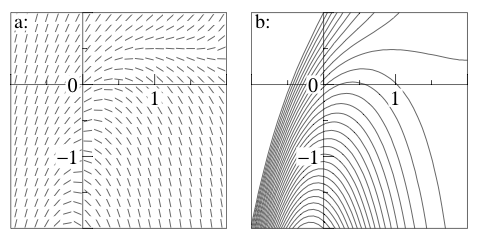One of the oldest differential equation in history!

### Backward error analysis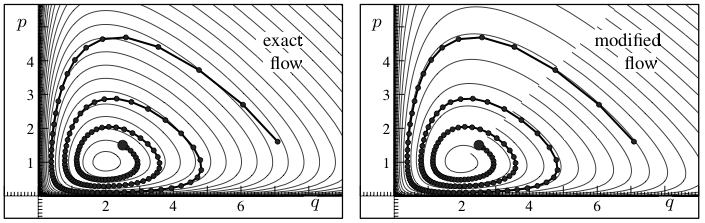An integrator maps a vector field to a vector field

## Integrators

Types: $$X_n$$ vector fields on $$\mathbb{R}^n$$

Integrator: map vf to vf $\phi \colon X \to X$ “Polymorphic in the dimension”

## Specifications?

$$R\colon \mathbb{R^n}\to\mathbb{R^m}$$

Induces a relation $$\simeq_R$$ by

$$f \simeq_R g \iff R'.f(x) = g(R(x))$$

Specification: $$f \simeq_R g\implies φ(f) \simeq_R φ(g)$$

Identity is the only possibility

## Relaxing specifications

[McLachlan, Modin, Munthe-Kaas, V. '14]

Only allow affine relations $$R$$.

The method is immune to:

• Change of origin, units, rotations, shearing
• Respects decoupled systems

Only possibility: Runge–Kutta methods

## Take home message?

New discipline: specification based numerical analysis

# Part 2: Spin systems

### Equation of motion

Newton:$\frac{d^2}{dt^2}q = -\nabla U(q)$

Differential equation: $\frac{d}{dt}q = p/m \quad \frac{d}{dt}p = -\nabla U(x)$

General form: $\frac{d}{dt}x = f(x)$

## Symplecticity

Variables are paired in $$(q,p)$$: sum of 2D planes

Symplecticity: the sum of projected areas is constant

## Symplectic integrator

An integrator which solves exactly another mechanical system

## Kepler problem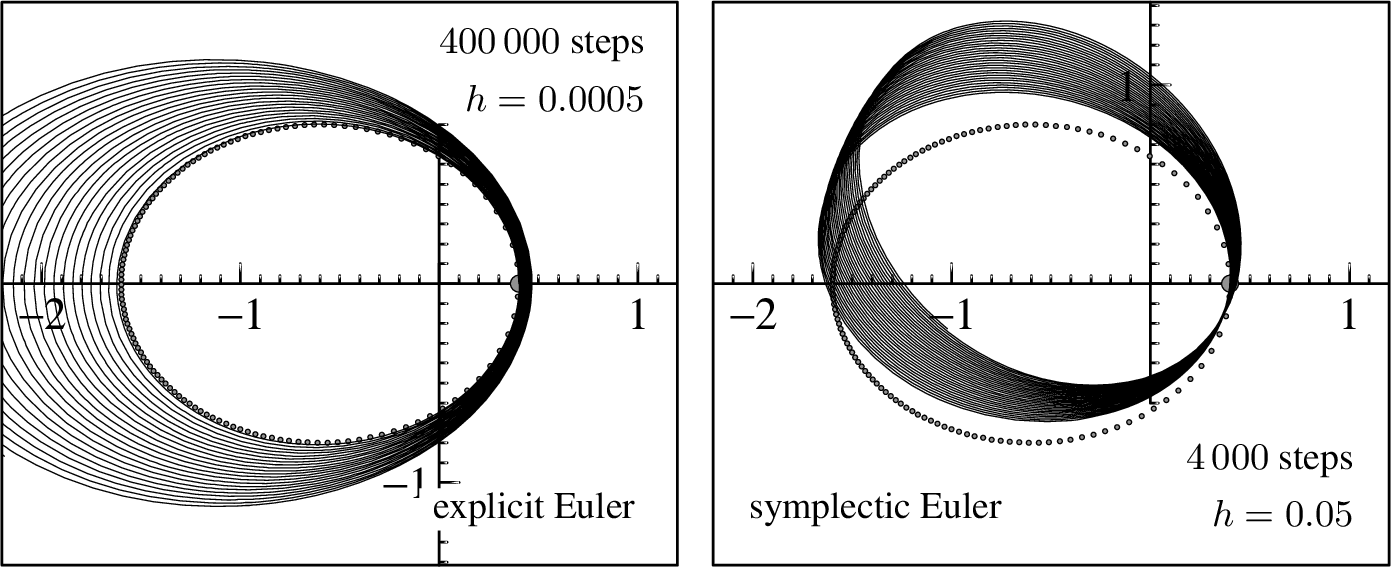## Leap-frog

aka Verlet$q_1 = q_{-1} -∆t^2\,\nabla U(q_0)$

Symplectic, uses the $$(q,p)$$ decomposition

## Midpoint

$x_1 = x_0 + ∆t\,f((x_0+x_1)/2)$

Symplectic, but does not use the $$(q,p)$$ decomposition. Magic!

## Spin systems

2D planes replaced by 2D spheres

### Hurricanes on gas planets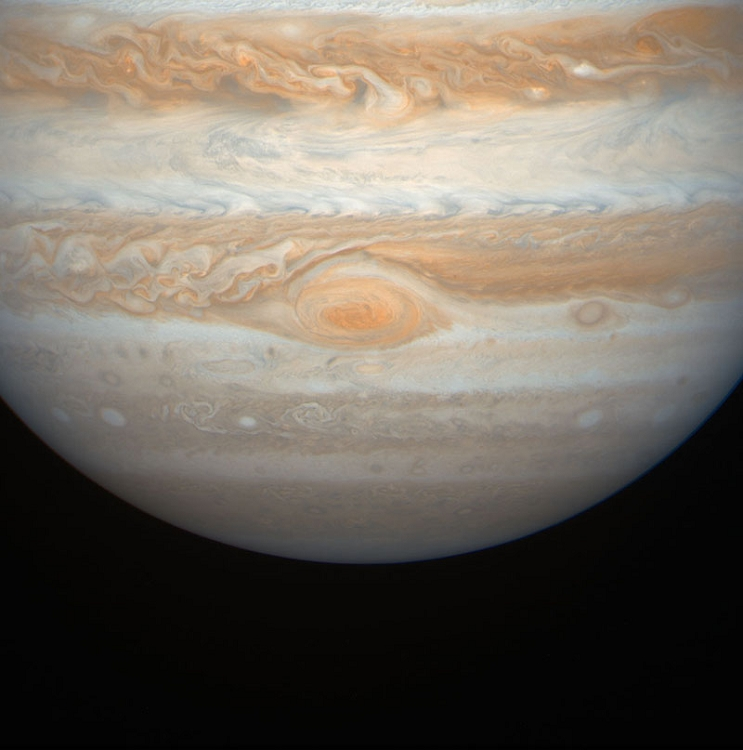## Hurricane interaction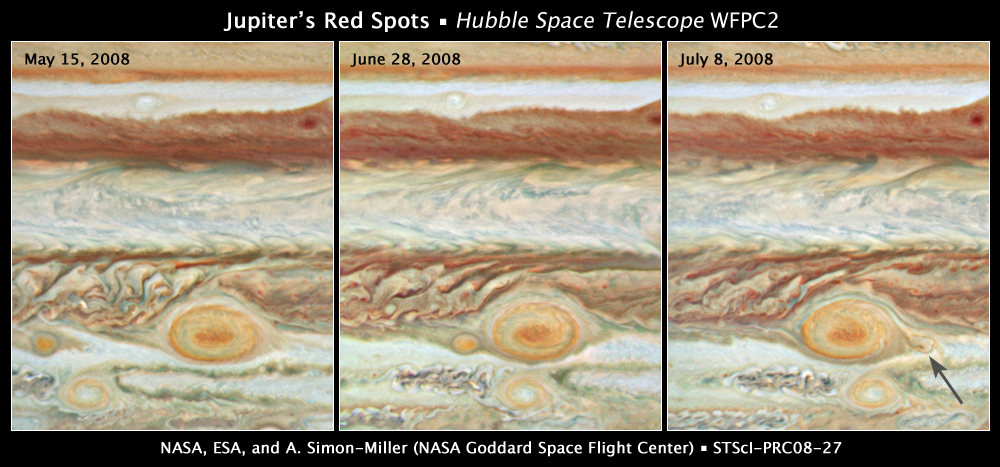## Point vortices

$\frac{d}{dt} r^i = \sum_{j\neq i} Γ^{j} \frac{r^i \times r^j}{\|r^j - r^i\|}$

$$r^i$$ position of i-th hurricane

$$Γ^i$$: “force” of the i-th hurricane

Symplectic system

## “Midpoint” on spheres

[McLachlan, Modin, V. '14]

$r_1 = r_0 + Δt \, f\Big(\frac{r_0+r_1}{\|r_0+r_1\|}\Big)$

Properties:

• Stays on the spheres
• Symplectic!

Note: proof of symplecticity has nothing to do with usual midpoint

## Simulation

[McLachlan, Modin, V. '14]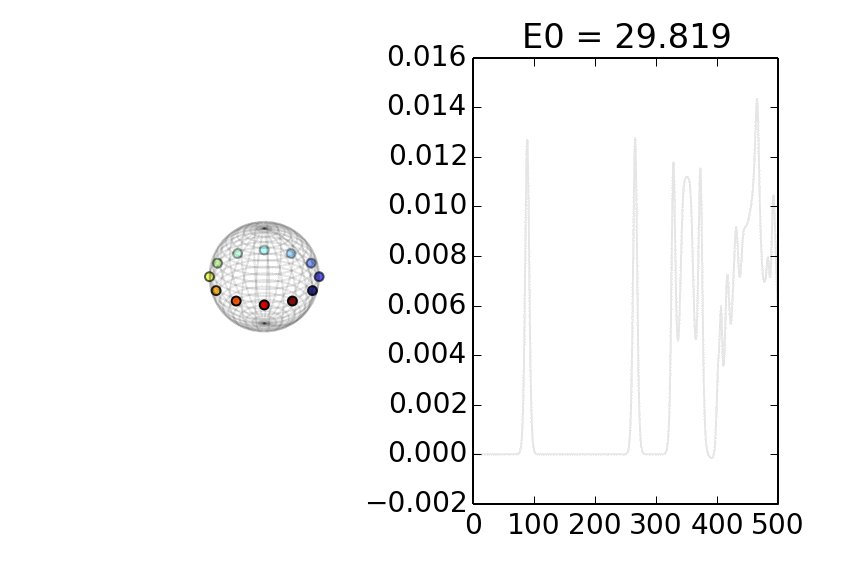## Conclusion

1. Runge–Kutta methods are the only affine compatible integrator
2. Symplectic “midpoint” method on sphere

# Thank You

### Bibliography

• “B-series methods are exactly the local, affine equivariant methods” with McLachlan, Modin, Munthe-Kaas
arXiv:1409.1019
• “Aromatic Butcher Series” with Munthe-Kaas
FoCM, arXiv:1308.5824 (2015)
• “Symplectic integrators for spin systems” with McLachlan, Modin
Phys. Rev. E., arXiv:1402.4114 (2014)
• “Discrete time Hamiltonian spin systems” with McLachlan, Modin
arXiv:1402.3334 (2014)